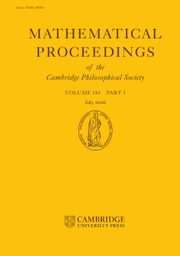Home
Hostname: page-component-7ccbd9845f-ktfbs Total loading time: 0.772 Render date: 2023-01-28T08:31:30.416Z Has data issue: true Feature Flags: { "useRatesEcommerce": false } hasContentIssue trueMathematical Proceedings of the Cambridge Philosophical Society

# Number fields without universal quadratic forms of small rank exist in most degrees

Published online by Cambridge University Press:  06 May 2022

## Abstract

We prove that in each degree divisible by 2 or 3, there are infinitely many totally real number fields that require universal quadratic forms to have arbitrarily large rank.

Type
Research Article

## Access options

Get access to the full version of this content by using one of the access options below. (Log in options will check for institutional or personal access. Content may require purchase if you do not have access.)

## Footnotes

The author was supported by Czech Science Foundation GAČR, grant 21-00420M, and by Charles University, projects PRIMUS/20/SCI/002 and UNCE/SCI/022.

## References

Bhargava, M.. On the Conway–Schneeberger fifteen theorem. Contemp. Math. 272 (1999), 2737.CrossRefGoogle Scholar
Bhargava, M. and Hanke, J.. Universal quadratic forms and the 290-theorem. Preprint.Google Scholar
Bhargava, M., Shankar, A. and Wang, X.. Squarefree values of polynomial discriminants I. Invent. Math. (to appear), https://arxiv.org/abs/1611.09806.Google Scholar
Blomer, V. and Kala, V.. Number fields without universal n-ary quadratic forms. Math. Proc. Camb. Phil. Soc. 159 (2015), 239252.Google Scholar
Blomer, V., Kala, V.. On the rank of universal quadratic forms over real quadratic fields. Doc. Math. 23 (2018), 1534.Google Scholar
Čech, M., Lachman, D., Svoboda, J., Tinková, M. and Zemková, K.. Universal quadratic forms and indecomposables over biquadratic fields. Math. Nachr. 292 (2019), 540555.Google Scholar
Chan, W. K., Kim, M.-H. and Raghavan, S.. Ternary universal integral quadratic forms. Japan. J. Math. 22 (1996), 263273.CrossRefGoogle Scholar
Chan, W. K. and Oh, B.-K.. Can we recover an integral quadratic form by representing all its subforms?. Preprint, https://arxiv.org/abs/2201.08957.Google Scholar
Deutsch, J. I.. Universality of a non-classical integral quadratic form over$\mathbb Q(\sqrt 5)$ . Acta Arith. 136 (2009), 229242.Google Scholar
DoleƝálek, M.. Subfields of number field extensions and quadratic forms. Bachelor’s thesis. Charles University (2022, to appear).Google Scholar
Earnest, A. G. and Khosravani, A.. Universal positive quaternary quadratic lattices over totally real number fields. Mathematika 44 (1997), 342347.CrossRefGoogle Scholar
Gras, M.-N.. Non monogénéité de l’anneau des entiers des extensions cycliques de$\mathbb{Q}$ de degré premier$l\geq 5$ . J. Number Theory 23 (1986), 347353.CrossRefGoogle Scholar
Hsia, J. S., Kitaoka, Y. and Kneser, M.. Representations of positive definite quadratic forms. J. Reine Angew. Math. 301 (1978), 132141.Google Scholar
Kala, V.. Universal quadratic forms and elements of small norm in real quadratic fields. Bull. Aust. Math. Soc. 94 (2016), 714.Google Scholar
Kala, V. and Svoboda, J.. Universal quadratic forms over multiquadratic fields. Ramanujan J. 48 (2019), 151157.Google Scholar
Kala, V. and Tinková, M.. Universal quadratic forms, small norms and traces in families of number fields. Int. Math. Res. Not. IMRN (to appear), https://arxiv.org/abs/2005.12312.Google Scholar
Kala, V. and Yatsyna, P.. Lifting problem for universal quadratic forms. Adv. Math. 377 (2021), 107497, 24 pp.CrossRefGoogle Scholar
Kalyanswamy, S.. Inverse Galois Problem for Totally Real Number Fields. Cornell University Mathematics Department Senior Thesis, 2012. http://pi.math.cornell.edu/files/Research/SeniorTheses/kalyanswamyThesis.pdf.Google Scholar
Kedlaya, K. S.. A construction of polynomials with squarefree discriminants. Proc. Amer. Math. Soc. 140 (2012), 30253033.Google Scholar
Kim, B. M.. Universal octonary diagonal forms over some real quadratic fields. Comment. Math. Helv. 75 (2000), 410414.Google Scholar
Kim, B. M.. Kim, M.-H. and Oh, B.-K.. A finiteness theorem for representability of quadratic forms by forms. J. Reine Angew. Math. 581 (2005), 2330.Google Scholar
Kim, B. M., Kim, M.-H. and Park, D.. Real quadratic fields admitting universal lattices of rank 7. J. Number Theory 233 (2022), 456466.CrossRefGoogle Scholar
Krásenský, J., Tinková, M. and Zemková, K.. There are no universal ternary quadratic forms over biquadratic fields. Proc. Edinb. Math. Soc. 63 (2020), 861912.Google Scholar
Maaß, H.. Über die Darstellung total positiver Zahlen des Körpers$R(\sqrt 5)$ als Summe von drei Quadraten. Abh. Math. Sem. Univ. Hamburg 14 (1941), 185191.Google Scholar
Neukirch, J.. Algebraic Number Theory (Springer-Verlag, Berlin, 1999).CrossRefGoogle Scholar
Schur, I.. Über die Verteilung der Wurzeln bei gewissen algebraischen Gleichungen mit ganzzahligen Koeffizienten. Math. Z. 1 (1918), 377402.Google Scholar
Shanks, D.. The simplest cubic number fields. Math. Comp. 28 (1974), 11371152.CrossRefGoogle Scholar
Siegel, C. L.. Sums of m th powers of algebraic integers. Ann. of Math. 46 (1945), 313339.CrossRefGoogle Scholar
Yatsyna, P.. A lower bound for the rank of a universal quadratic form with integer coefficients in a totally real field. Comment. Math. Helv. 94 (2019), 221239.Google Scholar

# Save article to Kindle

Note you can select to save to either the @free.kindle.com or @kindle.com variations. ‘@free.kindle.com’ emails are free but can only be saved to your device when it is connected to wi-fi. ‘@kindle.com’ emails can be delivered even when you are not connected to wi-fi, but note that service fees apply.

Find out more about the Kindle Personal Document Service.

Number fields without universal quadratic forms of small rank exist in most degrees
Available formats
×

# Save article to Dropbox

To save this article to your Dropbox account, please select one or more formats and confirm that you agree to abide by our usage policies. If this is the first time you used this feature, you will be asked to authorise Cambridge Core to connect with your Dropbox account. Find out more about saving content to Dropbox.

Number fields without universal quadratic forms of small rank exist in most degrees
Available formats
×

# Save article to Google Drive

To save this article to your Google Drive account, please select one or more formats and confirm that you agree to abide by our usage policies. If this is the first time you used this feature, you will be asked to authorise Cambridge Core to connect with your Google Drive account. Find out more about saving content to Google Drive.

Number fields without universal quadratic forms of small rank exist in most degrees
Available formats
×
×